Electron. J. Diff. Eqns., Vol. 2004(2004), No. 119, pp. 1-7.

### Semipositone-point boundary-value problems Nickolai Kosmatov

Abstract:
We study the-point nonlinear boundary-value problem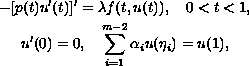where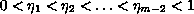,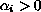for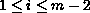and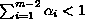,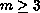. We assume that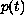is non-increasing continuously differentiable on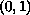and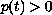on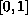. Using a cone-theoretic approach we provide sufficient conditions on continuous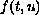under which the problem admits a positive solution.

Submitted April 23, 2004. Published October 10, 2004.
Math Subject Classifications: 34B10, 34B18.
Key Words: Green's function; fixed point theorem; positive solutions; multi-point boundary-value problem.

Show me the PDF file (204K), TEX file, and other files for this article.

 Nickolai Kosmatov Department of Mathematics and Statistics University of Arkansas at Little Rock Little Rock, AR 72204-1099, USA email: nxkosmatov@ualr.edu